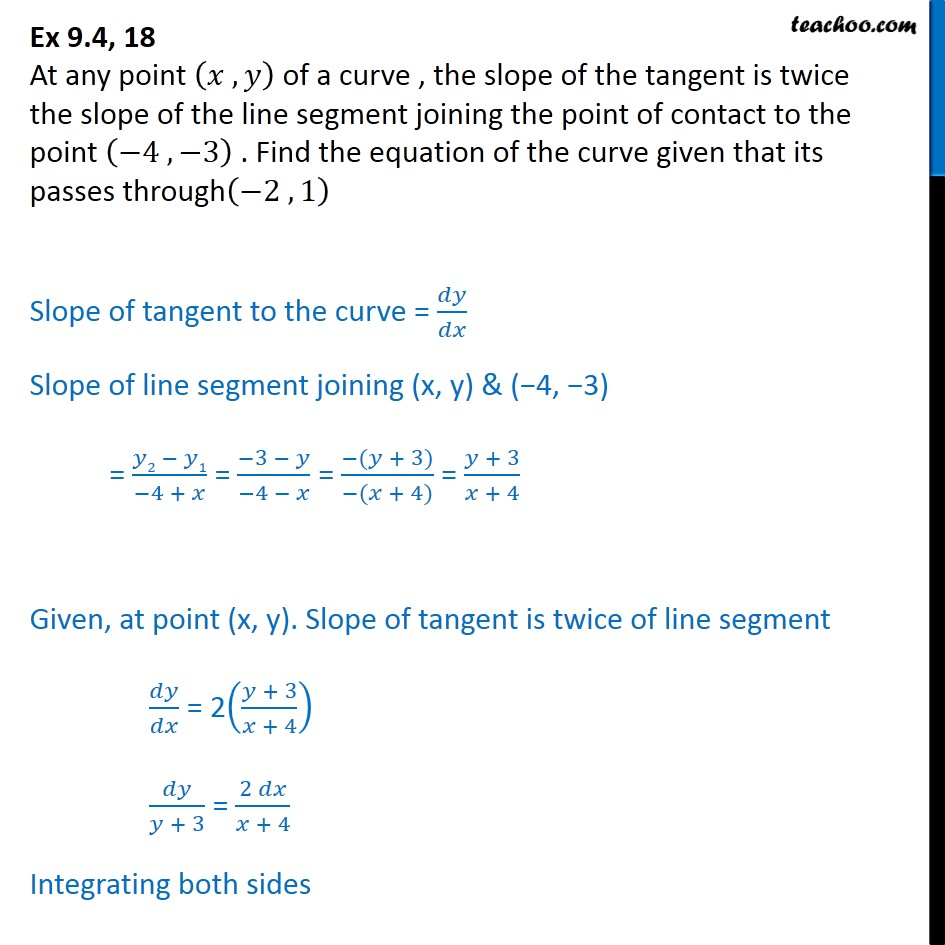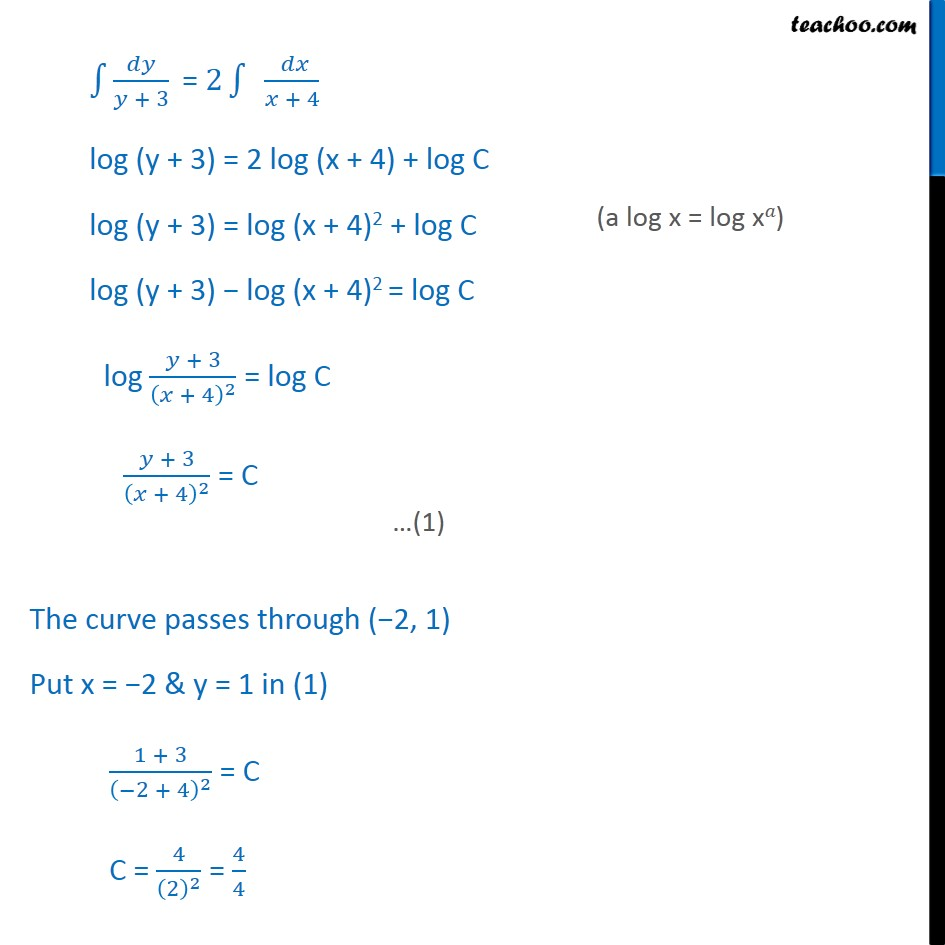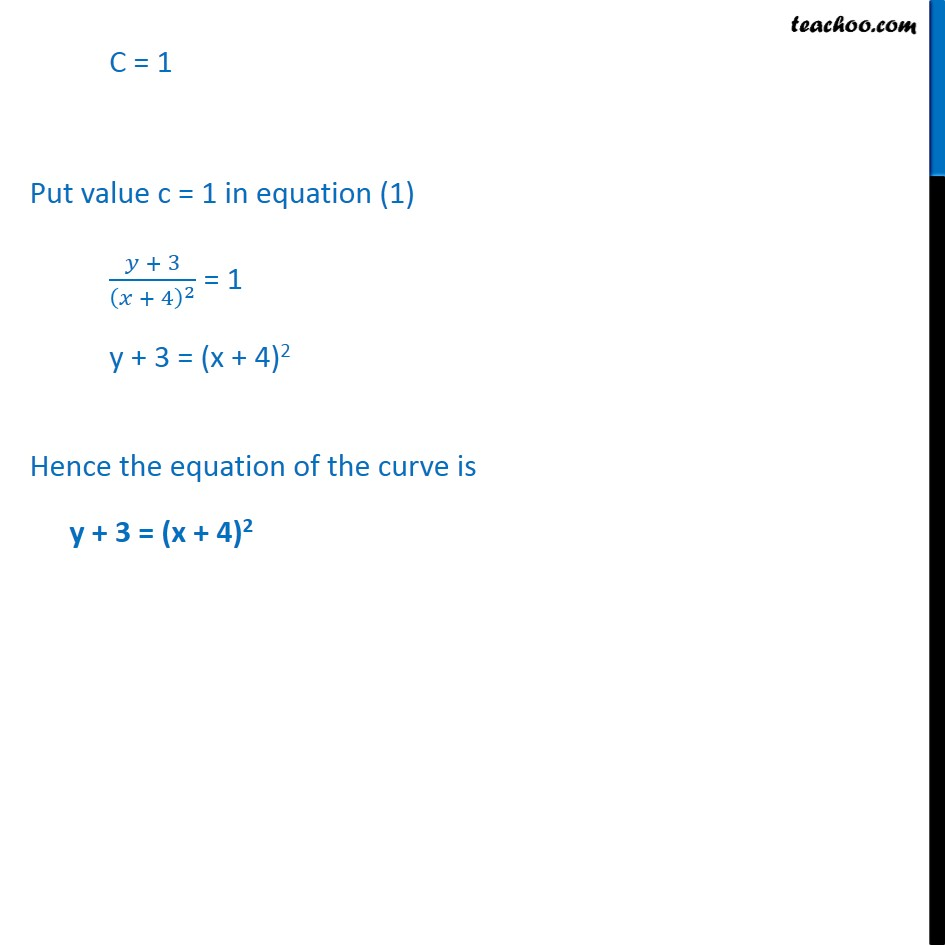Ex 9.3

Chapter 9 Class 12 Differential Equations
Serial order wiseLearn in your speed, with individual attention - Teachoo Maths 1-on-1 Class

### Transcript

Ex 9.3, 18 At any point , of a curve , the slope of the tangent is twice the slope of the line segment joining the point of contact to the point 4 , 3 . Find the equation of the curve given that its passes through 2 , 1 Slope of tangent to the curve = Slope of line segment joining (x, y) & ( 4, 3) = 2 1 4 + = 3 4 = ( + 3) ( + 4) = + 3 + 4 Given, at point (x, y). Slope of tangent is twice of line segment = 2 + 3 + 4 + 3 = 2 + 4 Integrating both sides + 3 = 2 + 4 log (y + 3) = 2 log (x + 4) + log C log (y + 3) = log (x + 4)2 + log C log (y + 3) log (x + 4)2 = log C log + 3 + 4 2 = log C + 3 + 4 2 = C The curve passes through ( 2, 1) Put x = 2 & y = 1 in (1) 1 + 3 2 + 4 2 = C C = 4 2 2 = 4 4 C = 1 Put value c = 1 in equation (1) + 3 + 4 2 = 1 y + 3 = (x + 4)2 Hence the equation of the curve is y + 3 = (x + 4)2